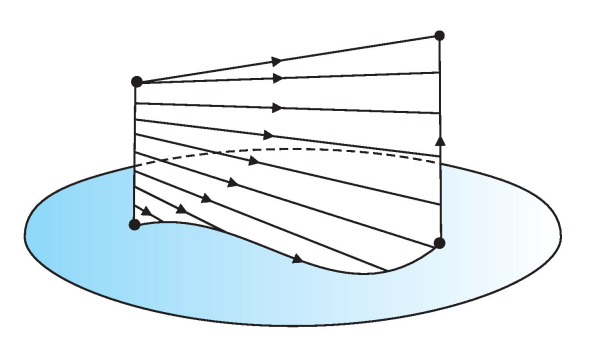Prove homotopic attaching maps give homotopy equivalent spaces by attaching a cell

Prove: If $f,g:S^{n-1} \to X$ are homotopic maps, then $X\sqcup_fD^n$ and $X\sqcup_gD^n$ are homotopy equivalent.

I think it can be proved by showing they are both deformation retracts of $X\sqcup_H(D^n\times I)$ where $H$ is the homotopy between $f$ and $g$.

However, I have difficult in proving that the deformation retracts are continuous map. In fact, I have difficulty in representing a map in quotient spaces like $X\sqcup_fD^n$. I think a map from $X\sqcup_fD^n$ to $W$ can be represented by two maps: $m_1: X\to W$, $m_2: D^n\to W$, where for $x\in S^{n-1}$, $m_1\circ f(x)=m_2\circ i(x)$.

Then I construct the deformation retract this way: $m_1: X\to X$. For $x\in H(S^{n-1},t)$, $m_1(x)=H(S^{n-1},0)$, otherwise $m_1(x)=x$.

$m_2: D^n\times I\to D^n\times {0}$: $m_2((D^n,t))=(D^n,0)$.

It is easy to verify that $m_1$ and $m_2$ define a map from $X\sqcup_H(D^n\times I)$ to $X\sqcup_fD^n$. As long as this is a continous map, obvioulsy then we find a deformation retract. But it seems such a map is not continous?

• Your idea is good. However, since you do not know anything about $X$, you will have to have $m_1={\rm id}_X$, since you do not really know any other continuous maps from $X$ to $X$ (constant maps aside). So $m_2$ will require more attention (we do know $D^n$!). Draw a picture for $n=1$ and go from there. – Carsten S Dec 16 '13 at 10:58

There is a retraction of $D^n\times I\twoheadrightarrow D^n×\{0\}\cup S^{n-1}×I$ defined via $$r(x,t)=\begin{cases} \left(\frac{2x}{2-t},\ 0\right) &\text{, if }t\le2(1-||x||) \\ \left(\frac x{||x||},2-\frac{2-t}{||x||}\right)&\text{, if }t\ge2(1-||x||) \end{cases}$$ It is easy to prove that this map is well-defined and continuous and a retraction. Then $$d:D^n×I×I\to D^n×I\\ d(x,t,s)=sr(x,t)+(1-s)(x,t)$$ is a homotopy between the identity and $r$, so $r$ is a deformation retraction. But then $(D^n×I)\cup_F X$ deformation retracts onto $(D^n×\{0\}\cup S^{n-1}×I)\cup_H X=(D^n×\{0\})\cup_f X$

Note that a pushout square ($A,X$, and $B$ are arbitrary spaces)$\$ gives rise to a pushout square$\$because the quotient map $q:X\sqcup B\to X\cup_f B$ induces a quotient map $q\times 1:X\times I\sqcup B\times I\to(X\cup_f B)\times I$.
This means that a pair of homotopies $F_t:X→Y$, $G_t:B→Y$, such that $F_ti=G_t f$ for all $t\in I$, induces a homotopy $H_t:X∪_f B→Y$
That's the reason why a deformation retraction on $D^n×I$ induces a deformation retraction on the pushout $(D^n×I)\cup_F X$

There is more general result: If $(X,A)$ is cofibered, then $X×I$ deformation retracts to $X×\{0\}\cup A×I$, so if $X$ is glued via two homotopic maps $f$ and $g$ to a space $B$, then $X\cup_f B$ and $X\cup_g B$ are homotopy equivalent.

• Is $r$ or $d$ a deformation retraction? Also can you elaborate more on why $(D^n×\{0\}\cup S^{n-1}×I)\cup_F X=(D^n×\{0\})\cup_f X$? I guess the result follows by using the same argument with deformation retracting the solid cylinder onto $(D^n×\{1\}\cup S^{n-1}×I)$ which in turn gives the identification space with $g$ as the attaching map correct? I suppose $F$ is the homotopy between $f$ and $g$? – Alp Uzman Jan 2 '16 at 16:54
• @A.AlpUzman: The space $(D^n×\{0\}∪S^{n−1}×I)∪_F X$ is homeomorphic to $(D^n×\{0\})∪_f X$ by the map sending $D^n×\{0\}$ and $X$ to itself and $S^{n-1}×I$ to $F(S^{n-1}×I)$, where $F$ is the homotopy from $f$ to $g$ (The asker used $H$ instead of $F$, I've edited my answer to fit the notation in the question). And yes, the result follows exactly by the argument you give. – Stefan Hamcke Jan 3 '16 at 16:36

This is also proved in Topology and Groupoids (as it was in the 1968 edition, "Elements of Modern Topology"); this has some pictures of the crucial mapping cylinder construction $$M(f) \cup X$$ which, if $$i: A \to X$$ is a cofibration, is a useful model of the adjunction space $$B \cup _f X$$ for $$f: A \to B$$. Here is a coloured picture of the homotopy as Fig 7.10:I just learned that the fact that both adjunction spaces are homotopy equivalent to each other can be seen as an immediate consequence of a general property:

Let $h\mathbf{Top}^B$ denote the homotopy category under $B$, the quotient category of $(B\downarrow\mathbf{Top})$ where we identify $f\sim g:i\to j$ if there is a homotopy $H:f\simeq g$ under $B$, that is $H(i\times 1)=j$. Let $\pi B^A$ denote the track groupoid whose objects are maps $A→B$ and whose arrows are homotopies $H:f\simeq g$ where $H$ and $K$ are identified if there's a continuous deformation between them which leaves $f$ and $g$ fixed.
The statement is that if $j:A\to X$ is a cofibration, there exists a contravariant functor $\beta$ from the track groupoid $\pi B^A$ to the category $h\mathbf{Cof}^B$, the full subcategory of $h\mathbf{Top}^B$ whose objects are cofibrations. This $\beta$ assigns to an $f:A→B$ the cofibration $j_f:B\to X\cup_f B$, and to a morphism $[\phi]:f→g$ the homotopy class $[k]$ of maps $j_g\to j_f$, where $k$ is induced by extending the homotopy $\phi:A→B$ to a homotopy $\Phi:X→X\cup_f B$ and setting $k=\Phi_1\cup j_f$.

You can find the proof in tom Dieck's Algebraic Topology where it is theorem $5.1.9$

Note that $[k]=\beta[\phi]$ is an isomorphisms by functoriality, hence a homotopy equivalence.

• People may like to compare the proof in tom Dieck's book with that in "Topology and Groupoids". They both use in this area the operation of a track groupoid. – Ronnie Brown Nov 15 '18 at 12:37